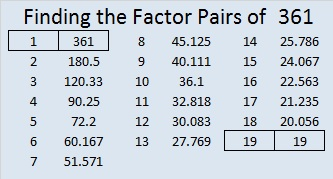# 1451 Star of Wonder

An important part of Mathematics is noticing patterns. I love it when mathematicians ask students, “What do you notice? What do you wonder?”

Those are questions you can ponder as you gaze on this star of wonder made from several different graphs.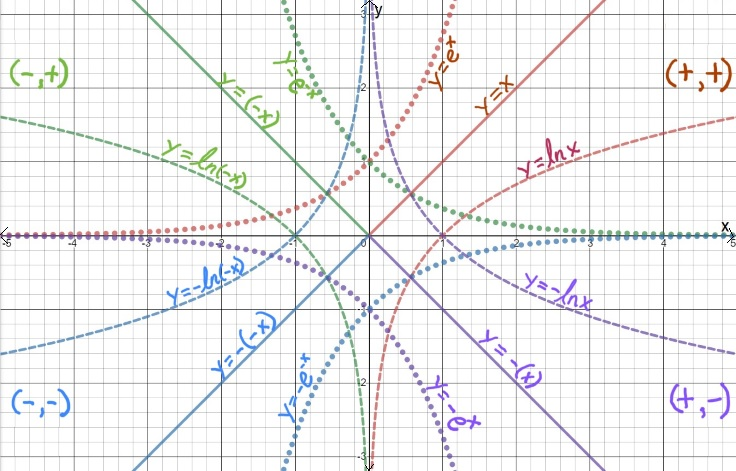To help distinguish the graphs, the dotted lines are exponential functions, the dashed lines are natural logarithm functions, and the solid lines are linear functions.

What do you notice? What do you wonder?

Now I’ll tell you a little bit about the post number, 1451:

• 1451 is a prime number.
• Prime factorization: 1451 is prime.
• 1451 has no exponents greater than 1 in its prime factorization, so √1451 cannot be simplified.
• The exponent in the prime factorization is 1. Adding one to that exponent we get (1 + 1) = 2. Therefore 1451 has exactly 2 factors.
• The factors of 1451 are outlined with their factor pair partners in the graphic below.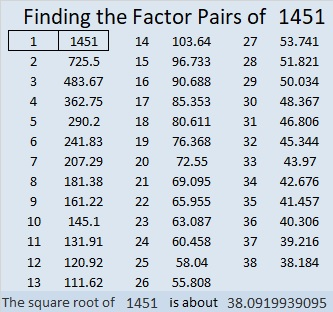How do we know that 1451 is a prime number? If 1451 were not a prime number, then it would be divisible by at least one prime number less than or equal to √1451. Since 1451 cannot be divided evenly by 2, 3, 5, 7, 11, 13, 17, 19, 23, 29 31, or 37, we know that 1451 is a prime number.

# 1301 Cool Patterns of Centered Square Numbers

1301 is a centered square number because it is the sum of two consecutive square numbers. It also happens to be exactly 100 more than the previous centered square number.

1301 is special because it is the sum of two consecutive squares, but it is not the only sum of two squares that is 100 more than another sum of two squares. Here is a chart of some of those squares with some other information. What patterns do you see?What role did the triangular numbers play on that chart? The pattern you see of 4, 12, 24, etc. is not new. Look at this chart of centered square numbers below. Below each centered square number (in blue) are related sums of squares. The difference of those related sums and the centered square above it follows that same 4, 12, 24, . . .  pattern.When you look just at centered square numbers, there is a different pattern for them as well. For example, if you know that 1301 is the 26th centered square number, then you can be sure that 1301 + 4(26) = 1405 will be the next centered square number.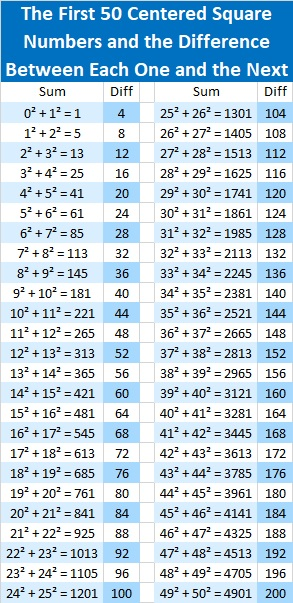When the chart above is “bent in half” so the list of centered square numbers begins at the lower left and ends at the lower right, there is yet another pattern. Look at the differences horizontally to see a cool pattern: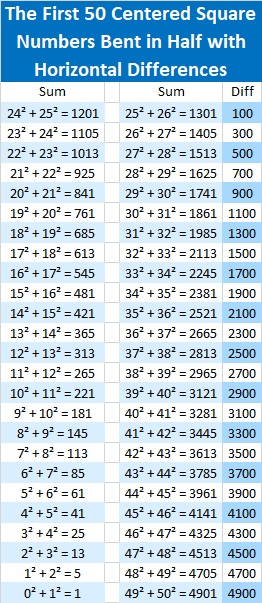I’ve made it clear that centered square numbers are made when consecutive square numbers are added, such as 26² + 25² = 1301, but what does one look like? Here’s a picture of 1301 tiny little squares arranged as a centered square.There are 100 tiny green squares around it because it is 100 more than the previous centered square number.

Here are some more facts about the number 1301:

• 1301 is a prime number and the first prime in a prime triplet.
• Prime factorization: 1301 is prime.
• The exponent of prime number 1301 is 1. Adding 1 to that exponent we get (1 + 1) = 2. Therefore 1301 has exactly 2 factors.
• Factors of 1301: 1, 1301
• Factor pairs: 1301 = 1 × 1301
• 1301 has no square factors that allow its square root to be simplified. √1301 ≈ 36.06938

How do we know that 1301 is a prime number? If 1301 were not a prime number, then it would be divisible by at least one prime number less than or equal to √1301 ≈ 36.1. Since 1301 cannot be divided evenly by 2, 3, 5, 7, 11, 13, 17, 19, 23, 29 or 31, we know that 1301 is a prime number.Every centered square number is a hypotenuse of a Pythagorean triple. For example,
primitive 51-1300-1301 is calculated from 26² – 25², 2(26)(25), 26² + 25²

Here’s another way we know that 1301 is a prime number: Since its last two digits divided by 4 leave a remainder of 1, and 26² + 25² = 1301 with 26 and 25 having no common prime factors, 1301 will be prime unless it is divisible by a prime number Pythagorean triple hypotenuse less than or equal to √1301 ≈ 36.1. Since 1301 is not divisible by 5, 13, 17, or 29, we know that 1301 is a prime number.

# 1252 What Do You Notice?

Remembering that 1250 = 25² + 25² from just a couple of days ago, I was struck when I noticed that 1252 = 24² + 26². I wondered if it was part of a pattern, so I made this chart. What do you think?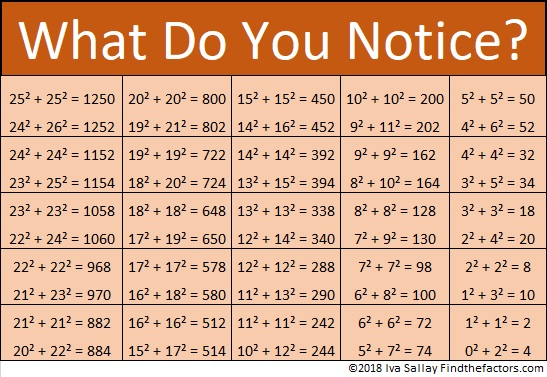When I thought about it more, I realized that perhaps it isn’t so remarkable. After all,
(n – 1)² + (n + 1)² = (n² – 2n +1) + (n² + 2n +1) = n² + n² – 2n + 2n + 1 + 1  = 2n² + 2
Nevertheless, I still like the pattern.

Here are some more facts about the number 1252:

• 1252 is a composite number.
• Prime factorization: 1252 = 2 × 2 × 313, which can be written 1252 = 2² × 313
• The exponents in the prime factorization are 2 and 1. Adding one to each and multiplying we get (2 + 1)(1 + 1) = 3 × 2  = 6. Therefore 1252 has exactly 6 factors.
• Factors of 1252: 1, 2, 4, 313, 626, 1252
• Factor pairs: 1252 = 1 × 1252, 2 × 626, or 4 × 313
• Taking the factor pair with the largest square number factor, we get √1252 = (√4)(√313) = 2√313 ≈ 35.38361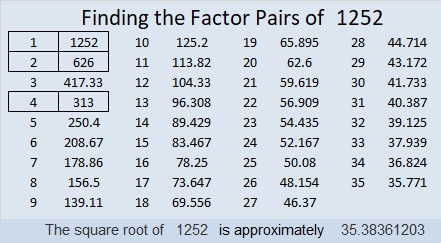Because 1252 = 26² + 24², it is the hypotenuse of a Pythagorean triple:
100-1248-1252 calculated from 26² – 24², 2(26)(24), 26² + 24²

100-1248-1252 is also 4 times (25-312-313)

# How I Knew Immediately that a Factor Pair of 1224 is . . .

12 = 3 × 4 and 24 is one less than 25. Those two facts helped me to know right away that 35² = 1225 and 34 × 36 = 1224. Study the patterns in the chart below and you will likely be able to remember all of the multiplication facts listed in it!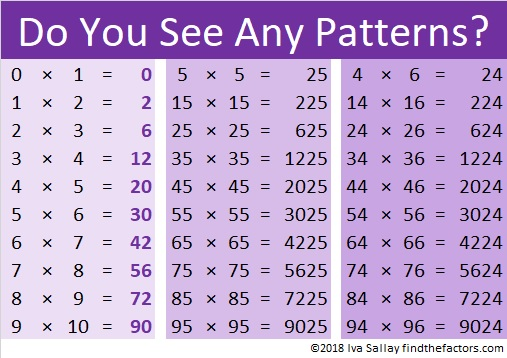a² – b² = (a – b)(a + b)
You may remember how to factor that from algebra class. Here when b = 1, it has a practical application that can allow you to amaze your friends and family with your mental calculating abilities!

I’ve only typed a small part of that infinite pattern chart. For example, if you know that 19 × 20 = 380, then you can also know that 195² = 38025 and 194 × 196 = 38024.

Also because of that chart, I know that 3.5² = 12.25 and 3.4 × 3.6 = 12.24
(Also (3½)² = 12¼, but 2½  × 4½ = 11¼ because 3-1 = 2, 3+1 = 4, 12-1 = 11
thus 2.5 × 4.5 = 11.25 and 2½  × 4½ = 11¼)

You could also let b = 2 so b² = 4. Then 25 – 4 = 21, and you could know facts like
33 × 37 = 1221 or 193 ×  197 = 38021

I hope you have a wonderful time being a calculating genius!

Now I’ll share some other facts about the number 1224:

• 1224 is a composite number.
• Prime factorization: 1224 = 2 × 2 × 2 × 3 × 3 × 17, which can be written 1224 = 2³ × 3² × 17
• The exponents in the prime factorization are 2, 3 and 1. Adding one to each and multiplying we get (3 + 1)(2 + 1)(1 + 1) = 4 × 3 × 2 = 24. Therefore 1224 has exactly 24 factors.
• Factors of 1224: 1, 2, 3, 4, 6, 8, 9, 12, 17, 18, 24, 34, 36, 51, 68, 72, 102, 136, 153, 204, 306, 408, 612, 1224
• Factor pairs: 1224 = 1 × 1224, 2 × 612, 3 × 408, 4 × 306, 6 × 204, 8 × 153, 9 × 136, 12 × 102, 17 × 72, 18 × 68, 24 × 51 or 34 × 36
• Taking the factor pair with the largest square number factor, we get √1224 = (√36)(√34) = 6√34 ≈ 34.98571When a number has so many factors, I often will make a forest of factor trees for that number, but today I just want us to enjoy this one tree for 34 × 36 = 1224.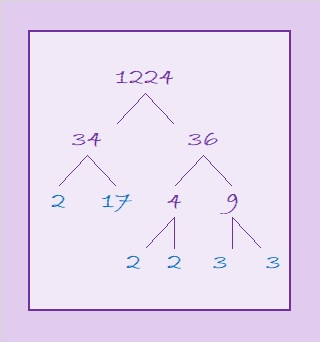1224 is also the sum of two squares:
30² + 18² = 1224

1224 is the hypotenuse of a Pythagorean triple:
576-1080-1224 which is (8-15-17) times 72
That triple can also be calculated from 30² – 18², 2(30)(18), 30² + 18²

293 + 307 + 311 + 313 = 1224 making 1224 the sum of four consecutive prime numbers.

# 378 Do You See a Pattern?

Most people know that 378 is a composite number because it’s obviously divisible by 2, but I know that 378 is a composite number because 18 x 21 = 378. I didn’t use a calculator or multiply 18 and 21 by hand. I know this multiplication fact because I can see a pattern, because 18 + 3 = 21 and because SUBTRACTING 2 from a number is easy.

“What is this pattern?” you ask. Here it is. Do you see the pattern, too?The pattern looks like this on the multiplication table: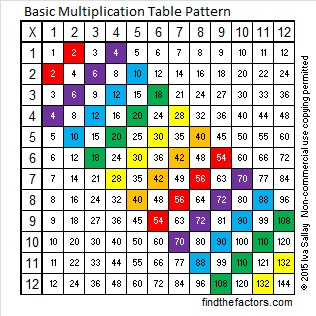So how did I know that 18 x 21 = 378? I knew because I can easily compute 19 x 20 = 380 in my head, and 380 – 2 = 378.

What is all the factoring information for 378?

• 378 is a composite number.
• Prime factorization: 378 = 2 x 3 x 3 x 3 x 7, which can be written 378 = 2 x (3^3) x 7
• The exponents in the prime factorization are 1, 3, and 1. Adding one to each and multiplying we get (1 + 1)(3 + 1)(1 + 1) = 2 x 4 x 2 = 16. Therefore 378 has exactly 16 factors.
• Factors of 378: 1, 2, 3, 6, 7, 9, 14, 18, 21, 27, 42, 54, 63, 126, 189, 378
• Factor pairs: 378 = 1 x 378, 2 x 189, 3 x 126, 6 x 63, 7 x 54, 9 x 42, 14 x 27, or 18 x 21
• Taking the factor pair with the largest square number factor, we get √378 = (√9)(√42) = 3√42 ≈ 18.442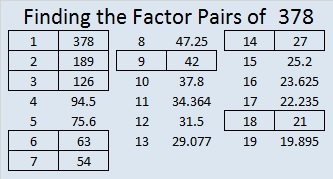# 361 Do You See a Pattern?

When 2^361 is divided by 361, the remainder is 116, not 2. That means that 361 is definitely a composite number. Its factors are listed at the end of this post.

361 isn’t used as often, but it is just as special as some of the numbers in the table below:The pattern can also be seen along the diagonals in this ordinary multiplication table:This pattern could be very helpful to students who are learning to multiply. I have seen plenty of students who knew 7 x 7 = 49, but couldn’t remember what 6 x 8 is.

Years after I learned the multiplication facts, I learned how to multiply binomials in an algebra class. I learned about the difference of two squares. In the example below one of the squares is n² and the other square is 1² which is equal to 1. I learned that the equation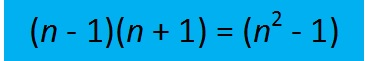is true for ALL numbers, but nobody pointed out any practical examples to make it more meaningful. The table at the top of the page contains twelve practical examples. Let’s see how you do applying it to products of a few larger numbers.

Sometimes we find easy ways to remember certain products like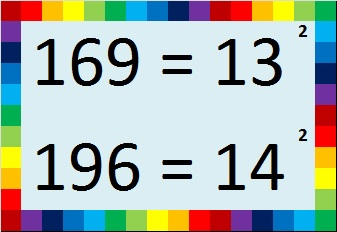We can use those products to help us remember other products easily by applying the difference of two squares. Try these: (Yes, you can easily do them without a calculator!)

• 13 x 13 = 169. How much is 12 x 14?
• 14 x 14 = 196. How much is 13 x 15?
• 20 x 20 = 400. How much is 19 x 21?
• If you know that the first ten powers of 2 are 2, 4, 8, 16, 32, 64, 128, 256, 512, 1024, then it’s easy to remember that 16 x 16 = 256. How much is 15 x 17?
• What if we go in the opposite direction…It isn’t too hard to multiply 18 x 20 in your head to get 360. How much is 19 x 19?
• 22 x 20 = 440 was also easy to find. How much is 21 x 21?
• 30 x 30 = 900. How much is 29 x 31?
• 100 x 100 = 10,000. How much is 99 x 101?Did you figure out what 361 has to do with this pattern? It is a perfect square just like 1, 4, 9, 16, and 25. Here is its factoring information:

• 361 is a composite number.
• Prime factorization: 361 = 19^2
• The exponent in the prime factorization is 2. Adding one we get (2 + 1) = 3. Therefore 361 has exactly 3 factors.
• Factors of 361: 1, 19, 361
• Factor pairs: 361 = 1 x 361 or 19 x 19
• 361 is a perfect square. √361 = 19